# Active Low SR Latch or Flip Flop: What is it? (Plus Truth Table)

## What is an Active Low SR Latch?

An active low SR latch (or active low SR Flip Flop) is a type of latch which is SET when S = 0(LOW). An active low SR latch is typically designed by using NAND gates. The logical circuit for a SR latch is shown below.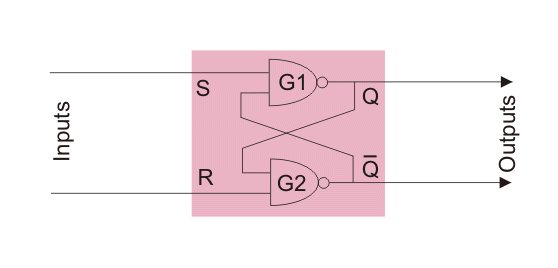In the above logic circuit if S = 0 and R = 1, Q becomes 1. Let us explain how.

• NAND gate always gives output 1 when at least one of the inputs is 0.
• So, when S is applied as 0 the output of gate G1 i.e. Q is 1 irrespective of the condition of second input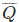to the gate.
• Now, Q is input of gate G2 so both the inputs of G2 become 1 as R is already 1. So output of G2 is now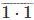or 0.
• So whatever may be the previous condition of Q, it always becomes Q = 1 and= 0 when S = 0 and R = 1. This is called SET condition of the latch.

In the above logic circuit if S = 1 and R = 0, Q becomes 0. Let us explain how.

• As we already said, a NAND gate always gives output 1 when at least one of the inputs is 0.
• So when R is applied as 0, the output of gate G2 i.e.is 1 irrespective of the condition of second input Q to the gate.
• So whatever may be the previous condition of, it always becomes 1 this 1 is then fed back to input of gate G1. As here S is already 1, both inputs of G1 are 1. Hence output of G1 i.e. Q will be 0. So Q = 0 and= 1 when, S = 1 and R = 0. This is called RESET condition of the latch.

In the above logic circuit if S = 1 and also R = 1, Q remains same as it was. Let us explain how.

• First suppose Q is previously 1.
• Now both inputs of G2 are 1 as R = 1 and Q = 1. So output of G2 i.e.isor 0.
• Now the inputs of G1 are 1 and 0 as R = 1 and= 0. So output of G1 i.e. Q isor 1.
• Now suppose Q is previously 0.
• Now the inputs of G2 are 1 and 0 as R = 1 and Q = 0. So output of G2 i.e.isor 1.
• Now both inputs of G1 are 1 as S = 1 and= 1. So output of G1 i.e. Q isor 0.
• So it is proved that Q remains same as it is when, S = 1 and also R = 1.

In the above logic circuit if S = 0 and also R = 0, the condition of Q is totally unpredictable. Let us explain how.

• First suppose Q is previously 0.
• Now both inputs of G2 are 0 as R = 0 and Q = 0. So output of G2 i.e.is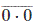or 1.
• Now the inputs of G1 are 0 and 1 as S=0 and= 1. So output of G1 i.e. Q is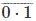or 1. That means Q is changed.
• Now Q is 1. So inputs of G2 are 0 and 1 as R = 0 and Q = 1. So output of G2 i.e.isor 1. That meansis unchanged.
• Now the inputs of G1 are 0 and 1 as S=0 and= 1. So output of G1 i.e. Q isor 1. That means Q is unchanged.

So, when both S and R are 0, it becomes unpredictable whether the value of output Q will be changed or unchanged. This condition of S R latch normally avoided.

## Active Low SR Latch Truth Table

The truth table for an active low SR flip flop (i.e. SR Latch) has been shown in the table below.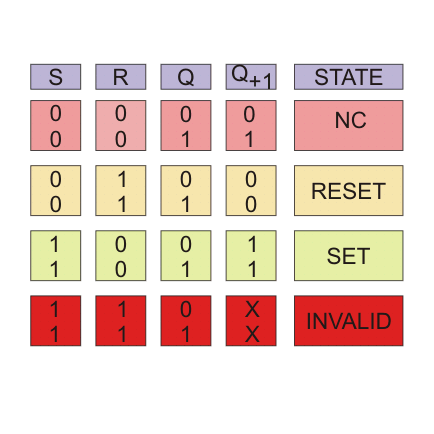There are also D Latches, JK Flip Flops, and Gated SR Latches. You can learn more about active low SR flip flops and other logic gates by checking out our full list of logic gates questions.

Want To Learn Faster? 🎓
Get electrical articles delivered to your inbox every week.
No credit card required—it’s 100% free.